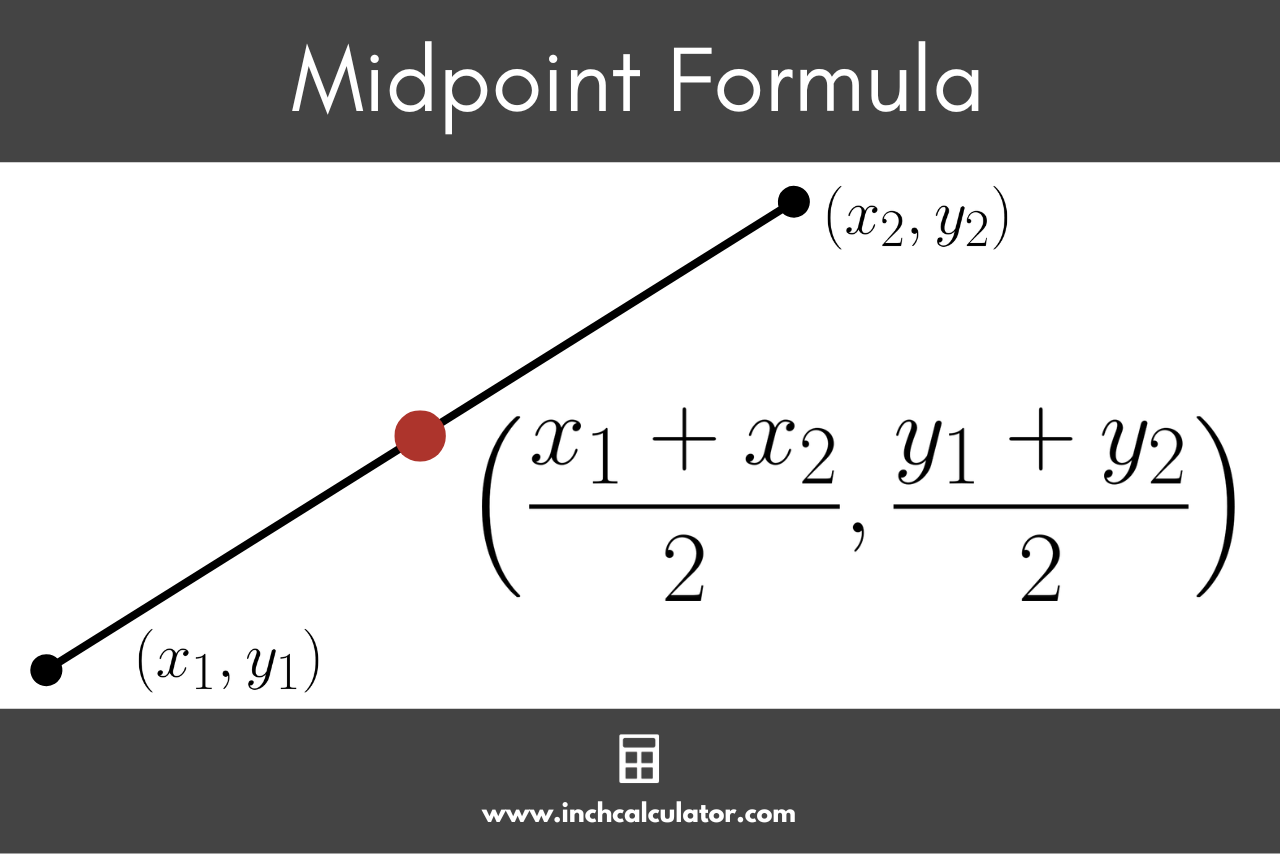# Midpoint Calculator

Use our midpoint calculator to find the midpoint of a line segment given its endpoints.

Point #1 Coordinates:
Point #2 Coordinates:

## Midpoint Coordinates:

(3, 5)

### Steps to Find the Midpoint

m = \left( \frac{x_{1} + x_{2}}{2}, \space \frac{y_{1} + y_{2}}{2} \right )

#### Substitute the endpoint values in the formula and solve

m = \left( \frac{1 + 5}{2}, \space \frac{3 + 7}{2} \right )
m = \left( \frac{6}{2}, \space \frac{10}{2} \right )
m = \left( 3, \space 5 \right )
Learn how we calculated this below

## How to Calculate the Midpoint of a Line Segment

The midpoint of a line segment is the point that lies exactly in the middle of the line, dividing it into two equal halves. This concept is widely used in mathematics, geometry, and even physics.

Calculating the midpoint is a straightforward process that involves finding the average of the coordinates of the two endpoints of the line segment.

You can calculate the midpoint of a line segment given the endpoints in a few simple steps.

### Step One: Identify the Endpoints

The first step to finding the midpoint of a line segment is to identify the coordinates of its two endpoints. The coordinates of the first endpoint are (x1, y1), and the coordinates for the second endpoint are (x2, y2).

### Step Two: Apply the Midpoint Formula

The midpoint formula allows you to find the coordinates of the midpoint of a line segment using the coordinates of its endpoints. The midpoint formula is similar to the formula to calculate the rise over run of a line, but is a little different.

The midpoint formula is:

m=\left ( \frac{x_{1}+x_{2}}{2}, \space \frac{y_{1}+y_{2}}{2} \right )

Thus the x-coordinate of the midpoint is equal to x1 plus x2 divided by 2, and the y-coordinate of the midpoint is equal to y1 + y2 divided by 2.You can also use our slope calculator to find the slope between these two points.

### Step Three: Verify the Result

To ensure the accuracy of your calculation, you can perform a quick check by measuring the distance between each endpoint and the midpoint. The distance from the first endpoint to the midpoint should be equal to the distance from the midpoint to the second endpoint.

You can use the distance formula to calculate the distance between two points.

d=\sqrt{(x_{2}-x_{1})^{2}+(y_{2}-y_{1})^{2}}

Then, verify that the distance from each endpoint to the midpoint is the same.

You might also be interested in our slope-intercept form calculator to learn how to express this line as an equation.